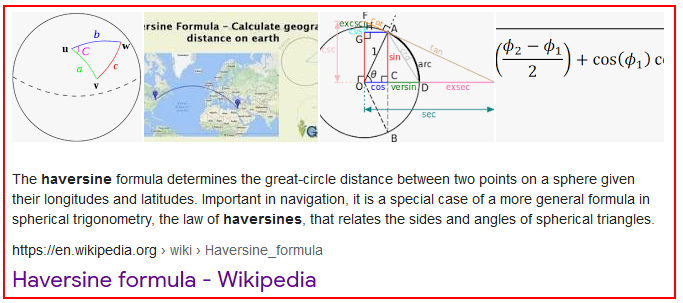# Formulas - How to calculate the distance between 2 points

We can calculate the distance between two longitude and latitude co-ordinates using a trigonometric calculation. It's a complex formula so in this post, we'll find out how exactly this works, including a verification of the result. We'll see how to perform this distance calculation in both kilometers and miles.

A question that arises occasionally is - how do I to calculate the distance (as the crow flies) between two points, based on longitude and latitude values?

A search for this question frequently return example formulas that mostly derive from a formula that Keith Whatling shares in his video beneath. I recommend watching this video.

This calculation contains a complex trigonometric equation. Whilst we can simply copy and paste the logic, it always makes good sense to understand how a formula works.

Therefore in this post, we'll examine more closely the maths behind this equation.

## What's the methodology behind this technique?

The methodology behind this technique relies on something called the Haversine formula. Wikipedia's article offers the following the succinct description – the "haversine formula determines the great-circle distance between two points on a sphere given their longitudes and latitudes”.In simple terms - to measure the distance between two points on Earth, we must apply a trigonometric function to account for the variance that the curvature of the Earth causes.

The Wikipedia and other mathematical based articles provide a full explanation of haversine formula but for our purposes, we can distil it down to the following pseudocode.

`lat1 = <latitude value of point A>lon1 = <longitude value of point A>lat2 = <latitude value of point B>lon2 = <longitude value of point B>r = 6371 #radius of Earth (KM)p = 0.017453292519943295  #Pi/180a = 0.5 - cos((lat2-lat1)*p)/2 + cos(lat1*p)*cos(lat2*p) * (1-cos((lon2-lon1)*p)) / 2d = 2 * r * asin(sqrt(a)) #2*R*asin`
The code above refers to the latitude and longitude of points A and B, expressed as lat1, lon1, lat2, and lon2.

The final value d, represents the distance between points A and B in kilometers.

There is also a dependency on two constants:
• p = pi divided by 180 degrees
• r = the approximate radius of the Earth (6371 km)

## How to calculate the distance with Power Apps

Taking our pseudocode, we can now build a Power Apps implementation of the formula. To demonstrate, we'll calculate the distance between London and Manchester:

• London = Latitude: 51.509865,   Longitude: -0.118092
• Manchester = Latitude: 53.483959,   Longitude: -2.244644

`With(    {        r: 6371,        p: (Pi() / 180),        latA: 51.509865,        lonA: -0.118092,        latB: 53.483959,        lonB: -2.244644    },    (2 * r) *     Asin(Sqrt(0.5 - Cos((latA - latB) * p)/2 + Cos(latB * p) * Cos(latA * p) *     (1 - Cos((lonA - lonB) * p)) / 2)))`

## Verifying our result

To provide some verification of our result, we'll compare our calculation against the result of an online calculator. We'll use the site that Keith mentions:This indicates a distance of 262.5km.

Our formula in Power Apps produces the same result.## Refinements - Rounding the Distance to 2 decimal places

Taking our base formula, we can adapt it as required. A typical requirement is to round the value, and we can do this by calling the round function like so:

`//Distance between 2 points in kilometers to 2 decimal placesWith(    {        r: 6371,        p: (Pi() / 180),        latA: 51.509865,        lonA: -0.118092,        latB: 53.483959,        lonB: -2.244644    },    Round(         (2 * r) *             Asin(Sqrt(0.5 - Cos((latA - latB) * p)/2 + Cos(latB * p) * Cos(latA * p) *             (1 - Cos((lonA - lonB) * p)) / 2)),         2   ))`

## How to calculate the distance between 2 points in miles

Our formula is based on the hardcoded value of the approximate radius of the earth in kilometres. To use a different unit of measurement, we can substitute the radius value as necessary.

To measure the distance in miles, we can substitute 6371km with the equivilant value in miles, 3958.8 miles.

`//Distance between 2 points in milesWith(    {        r: 3958.8,        p: (Pi() / 180),        latA: 51.509865,        lonA: -0.118092,        latB: 53.483959,        lonB: -2.244644    },    (2 * r) *     Asin(Sqrt(0.5 - Cos((latA - latB) * p)/2 + Cos(latB * p) * Cos(latA * p) *     (1 - Cos((lonA - lonB) * p)) / 2)))`
This formula produces the verifiable result of 163 miles, as shown beneath.## Conclusion

The Haversine formula enables us to calculate the distance between two points. This post described a how to perform this calculation in Power Apps in both kilometers and miles, including a verification of the result.